/* styles */
 table div table+table+table+table div table{width:100%;padding:0}table div table+table+table+table div table img{width:96.23%;padding:0;float:none}table div table+table+table+table div table td{width:100%;padding:0 1.88% 18px}/* styles */# Upcoming Important Dates

Monday, Monday 3 - Friday, May 7 - Teacher Appreciation Week
Friday, May 7 - First Friday Virtual Gathering
Friday, May 14 - Virtual Annual NCP Fundraiser

## News from the Counseling Office

▪ Health and Wellness Education Sessions
▪ Breaking Down Barriers Conference
▪ Class of 2021 Spring Checklist
▪ Apprenticeships
▪ Summer Enrichment/Pre-College Programs
▪ Chicago Teachers Union Scholarship
 ▪ Health and Wellness Education Sessions
 ▪ Breaking Down Barriers Conference
 ▪ Class of 2021 Spring Checklist
 ▪ Apprenticeships
 ▪ Summer Enrichment/Pre-College Programs
 ▪ Chicago Teachers Union Scholarship

## Sports, Yearbook & General School News

▪ NCP Admin Team to Send Weekly Updates to Parents on Fridays via SchoolCNXT and via Email
▪ NCP Sports Schedule for This Week
▪ Update on Outdoor Attendance
▪ NCP Car Magnets and Window/Yard Signs Available
▪ Order Your 2020-2021 NCP Yearbook
▪ Ways for Parents to Stay in Touch/Volunteer/Support NCP/Social Media Links
 ▪ NCP Admin Team to Send Weekly Updates to Parents on Fridays via SchoolCNXT and via Email
 ▪ NCP Sports Schedule for This Week
 ▪ Update on Outdoor Attendance
 ▪ NCP Car Magnets and Window/Yard Signs Available
 ▪ Order Your 2020-2021 NCP Yearbook
 ▪ Ways for Parents to Stay in Touch/Volunteer/Support NCP/Social Media Links
 table div table+table+table+table+table+table+table div table{width:100%;padding:0}table div table+table+table+table+table+table+table div table img{width:96.23%;padding:0;float:none}table div table+table+table+table+table+table+table div table td{width:100%;padding:0 1.88% 18px}/* styles */## Commencement 2021

 /* styles */ We are in the process of finalizing a contract with a venue and will share the date, time, and location of graduation with our senior families and students as soon as we have a signed contract. As previously stated, we can tell you the ceremony will occur outside and will adhere to the most current guidelines provided to us by CPS and the IDPH. As always, we appreciate your support and patience.
 table div table+table+table+table+table+table+table+table+table+table div table{width:100%;padding:0}table div table+table+table+table+table+table+table+table+table+table div table img{width:96.23%;padding:0;float:none}table div table+table+table+table+table+table+table+table+table+table div table td{width:100%;padding:0 1.88% 18px}/* styles */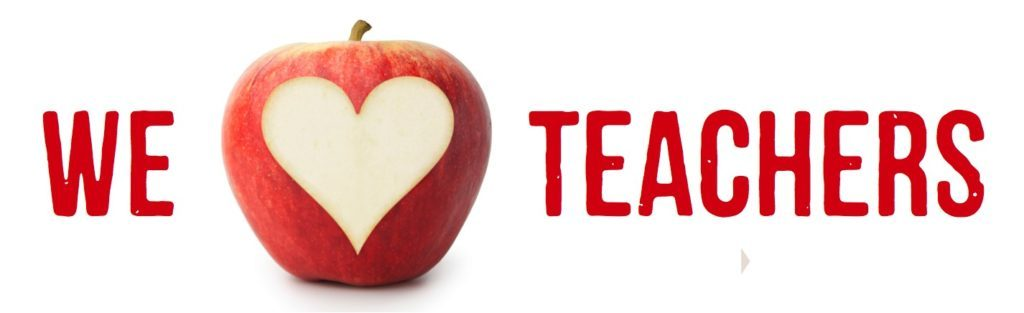## Teacher Appreciation Week

 /* styles */ Hi Parents! This week, May 3rd through May 7th is Teacher Appreciation week. We would love to honor our dedicated teachers and staff and all the hard work they have done during these unprecedented times over the past year. We would like to virtually show a little appreciation towards the teachers by compiling a collection of messages of teacher/staff appreciation and then have Friends of Northside email the faculty/staff the collection. Email your message to info@friendsofnorthside.org. The deadline for collecting the messages is Wednesday, May 5th. Please let us know if you want your name attached to your message or if you want your message to be anonymous. Thank you!
 table div table+table+table+table+table+table+table+table+table+table+table+table+table+table div table{width:100%;padding:0}table div table+table+table+table+table+table+table+table+table+table+table+table+table+table div table img{width:96.23%;padding:0;float:none}table div table+table+table+table+table+table+table+table+table+table+table+table+table+table div table td{width:100%;padding:0 1.88% 18px}/* styles */# First Friday Virtual Gathering

Friday, May 7
9:00 am Zoom Meeting

Each month, NCP parents and guardians are invited to get together for a monthly coffee gathering. This month, join fellow NCP parents online. Stay for as long as your schedule allows.

Meeting ID: 848 7451 7349
Passcode: 336446
One tap mobile
+13126266799,,84874517349#,,,,336446# US (Chicago)
+19294362866,,84874517349#,,,,
336446# US (New York)

+1 312 626 6799 US (Chicago)

 table div table+table+table+table+table+table+table+table+table+table+table+table+table+table+table+table div table{width:100%;padding:0}table div table+table+table+table+table+table+table+table+table+table+table+table+table+table+table+table div table img{width:96.23%;padding:0;float:none}table div table+table+table+table+table+table+table+table+table+table+table+table+table+table+table+table div table td{width:100%;padding:0 1.88% 18px}/* styles */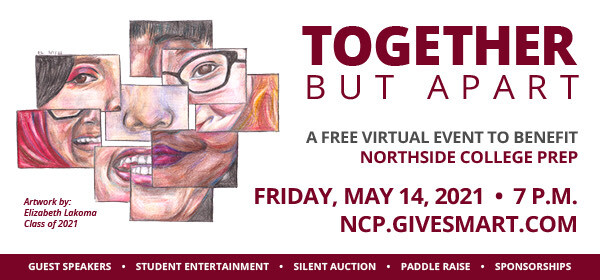## Only Two Weeks Left to Register for the Virtual Spring Benefit!

 /* styles */ Our year is coming to a close, and while we all can't yet celebrate in person as a community, we can be "Together But Apart" at our virtual spring benefit on Friday, May 14th at 7pm! This event will help raise funds for school programs not covered in the traditional CPS budget. Please support our school and celebrate our amazing kids. REGISTER TODAY!!! You won't want to miss special moments from Northside's students, teachers and special appearances by community leaders. The event is FREE to attend so we hope to "see" you there.

## Become a Parent or Family Sponsor!

 /* styles */ It's not too late to become a Parent or Family Sponsor. Sponsorship levels are at \$250, \$500, \$750, \$1000, \$1500, \$2000 and \$4000. Review page two of the above click through for various sponsorship level benefits. You may pay for sponsorship via check or choose to register online via credit card at ncp.givesmart.com If you have any questions, please email: Linda Weil-Silk at lindaweil-silk@hotmail.com

## Contribute a Gift Card from your Favorite Restaurant to the Auction!

 table div table+table+table+table+table+table+table+table+table+table+table+table+table+table+table+table+table+table+table+table+table+table+table+table+table+table+table div table{width:100%;padding:0}table div table+table+table+table+table+table+table+table+table+table+table+table+table+table+table+table+table+table+table+table+table+table+table+table+table+table+table div table img{width:96.23%;padding:0;float:none}table div table+table+table+table+table+table+table+table+table+table+table+table+table+table+table+table+table+table+table+table+table+table+table+table+table+table+table div table td{width:100%;padding:0 1.88% 18px}/* styles */## National Decision Day and Graduation Requirements

 table div table+table+table+table+table+table+table+table+table+table+table+table+table+table+table+table+table+table+table+table+table+table+table+table+table+table+table+table+table+table div table{width:100%;padding:0}table div table+table+table+table+table+table+table+table+table+table+table+table+table+table+table+table+table+table+table+table+table+table+table+table+table+table+table+table+table+table div table img{width:96.23%;padding:0;float:none}table div table+table+table+table+table+table+table+table+table+table+table+table+table+table+table+table+table+table+table+table+table+table+table+table+table+table+table+table+table+table div table td{width:100%;padding:0 1.88% 18px}/* styles */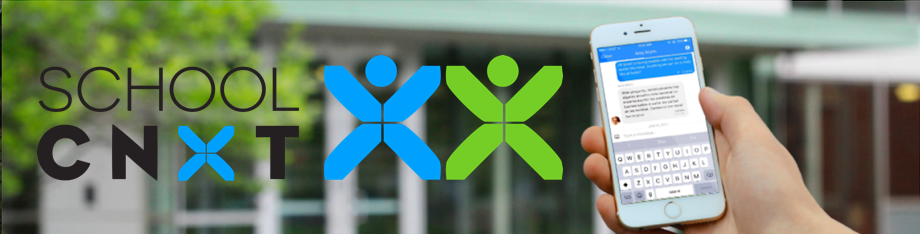# NCP Admin Team to Send Weekly Updates to Parents on Fridays via SchoolCNXT and via Email

From now on, NCP will be sending a consolidated update to parents every Friday with important information. Parents should be on the lookout for these updates, which will be posted via School CNXT and emailed to families.

You can gain access by using this tool on Northside's website.

If you are having problems with SchoolCNXT, please contact the main office from 7:30 am - 3:15 pm at 773-534-3954, or you can email the NCP school clerk, Mary Grasz, at mtgrasz@cpu.edu.

 table div table+table+table+table+table+table+table+table+table+table+table+table+table+table+table+table+table+table+table+table+table+table+table+table+table+table+table+table+table+table+table+table+table div table{width:100%;padding:0}table div table+table+table+table+table+table+table+table+table+table+table+table+table+table+table+table+table+table+table+table+table+table+table+table+table+table+table+table+table+table+table+table+table div table img{width:96.23%;padding:0;float:none}table div table+table+table+table+table+table+table+table+table+table+table+table+table+table+table+table+table+table+table+table+table+table+table+table+table+table+table+table+table+table+table+table+table div table td{width:100%;padding:0 1.88% 18px}/* styles */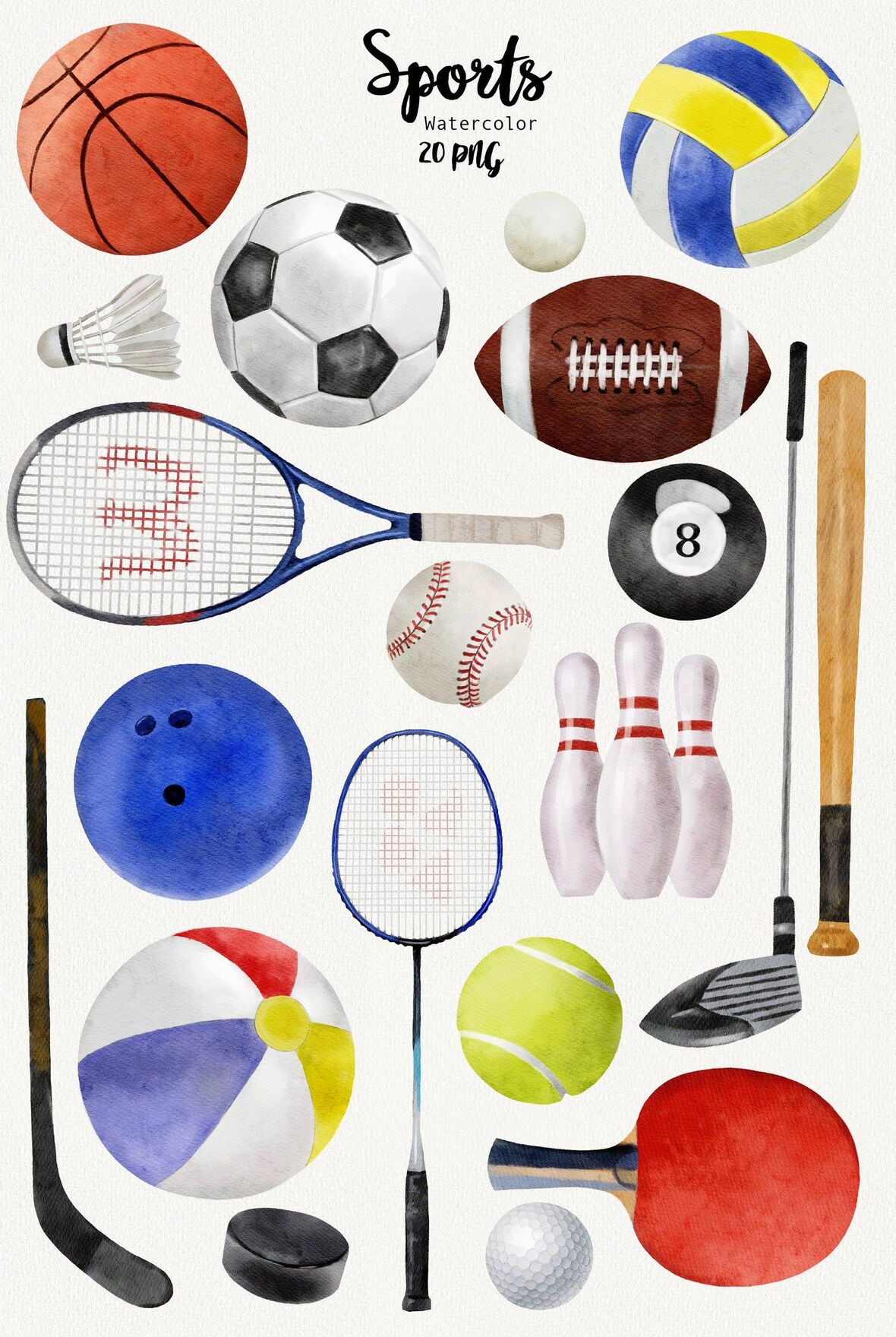# NCP Sports Schedule for This Week

Boys Volleyball

▪ Wednesday, May 5 vs. Mather @ NCP at 5:00 pm
▪ Friday, May 7 vs. Westinghouse @ NCP at 5:00 pm

Girls Soccer
▪ Tuesday, May 4 vs. Mather @ NCP (V at 4:30 pm; JV at 6:15 pm)
▪ Thursday, May 6 vs. Lakeview (away) (V at 4:30 pm; JV at 6:15pm)
▪ Saturday, May 8 vs. Jones @ NCP (V at 9:00 am; JV at 1:45 am)

Boys Baseball
▪ Monday, May 3 vs. Von Steuben (away/NEIU Field) at 4:30 pm
▪ Wednesday, May 5 vs. Von Steuben (home/River Park) at 4:30 pm
▪ Friday, May 7 vs. Whitney Young (away) at 7:00 pm
▪ Saturday, May 8 vs. Ag Science (away) at 4:45 pm

Girls Water Polo
▪ Monday, May 3 vs. Juarez @ NCP at 5:00 pm
▪ Wednesday, May 5 vs. Curie @ NCP at 5:00 pm
▪ Thursday, May 6 vs. Amundsen @ NCP at 5:00 pm

Girls Softball
▪ Monday, May 3 vs. Lane Tech (away) at 4:30pm (V and JV)
▪ Wednesday, May 5 vs. Brooks @ NCP (V at 4:30 pm)
▪ Friday, May 7 vs. Kennedy (away) (V at 4:30 pm)

Boys Lacrosse
▪ Wednesday, May 5 vs. Whitney Young @ NCP (V at 5:00 pm)
▪ Saturday, May 8 vs. Urban Prep @ NCP (V at 2:30 pm)

Girls Lacrosse
▪ Tuesday, May 4 vs. Lincoln Park (away) (V at 5:00 pm)
▪ Friday, May 7 vs. Whitney Young @ NCP (V at 5:00 pm)
▪ Saturday, May 8 vs. Solorio (away) (V at 11:30 am)

Boys Tennis
▪ Tuesday, May 4 vs. Lincoln Park @ NCP (V at 5:00 pm)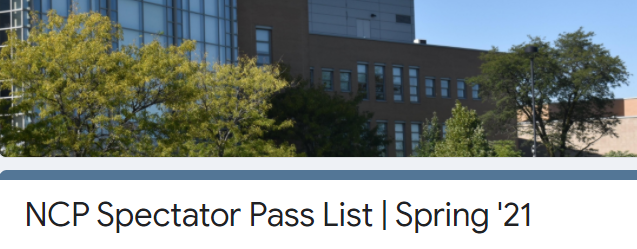# Update on Outdoor Attendance

At this time, CPS is not allowing spectators for indoor sports, however, limited spectators will be allowed for outdoor activities.

Per CPS guidelines: For games held outdoors, student-athletes and coaches may add the names of no more than two spectators to an approved pass list.

Spectators are restricted to family members only (i.e., parent/guardian, grandparent, sibling). Schools may ONLY allow entrance to individuals listed on the APPROVED pass list.

If you would like the opportunity to attend your NCP athlete's outdoor games, please identify the names of TWO family members for the NCP Spectator Pass List by completing this form.

Any spectators NOT on this list will NOT be allowed admission to NCP games.

In addition to this list, spectators will also need to:

1. Complete the required CPS Health Screener BY 2:00 pm on weekdays and 8:45 am on weekends.
2. Pass a temperature check.
3. Wear a CFC (cloth face covering or mask) at all times.
4. Maintain social distancing of 6 ft at all times with spectators from different households.
 1 Complete the required CPS Health Screener BY 2:00 pm on weekdays and 8:45 am on weekends.
 2 Pass a temperature check.
 3 Wear a CFC (cloth face covering or mask) at all times.
 4 Maintain social distancing of 6 ft at all times with spectators from different households.
 table div table+table+table+table+table+table+table+table+table+table+table+table+table+table+table+table+table+table+table+table+table+table+table+table+table+table+table+table+table+table+table+table+table+table+table+table+table div table{width:100%;padding:0}table div table+table+table+table+table+table+table+table+table+table+table+table+table+table+table+table+table+table+table+table+table+table+table+table+table+table+table+table+table+table+table+table+table+table+table+table+table div table img{width:96.23%;padding:0;float:none}table div table+table+table+table+table+table+table+table+table+table+table+table+table+table+table+table+table+table+table+table+table+table+table+table+table+table+table+table+table+table+table+table+table+table+table+table+table div table td{width:100%;padding:0 1.88% 18px}/* styles */# Supporting the Whole Child: Health and wellness education sessions to better support youth

## A series of virtual supports for CPS parents and caregivers

Time: Every Monday at 4:00 pm

The CPS Office of Student Health and Wellness and the Office of Family and Community Engagement are hosting educational sessions for CPS parents and caregivers on issues that affect youth health. Attend upcoming sessions:
May 3 - Sexual Health: STIs & Sexual Rights
May 10 - Sexual Health: Contraception & Reproductive Health

Sessions will have live Spanish interpretation. Request additional languages via the registration link.

For questions email OSHW@cps.edu.

# Apoyando A Los NIÑO:Sesiones de educación sobre salud y bienestar para brindar un mejor apoyo a los jóvenes

## Una serie de apoyos virtuales para padres y tutores de CPS

Todos los lunes a las 4:00 pm CT
Registrarse clic aquí
Volantes clic aquí

La Oficina de Salud y Bienestar Estudiantil de CPS y la Oficina de Participación Familiar y Comunitaria están organizando sesiones educativas para padres y tutores de CPS sobre temas que afectan la salud de los jóvenes. Asista a las próximas sesiones:
3 de mayo - Salud sexual: ITS y derechos sexuales
10 de mayo - Salud sexual: anticoncepción y salud reproductiva
Las sesiones contarán con interpretación en vivo al español.

Si tiene preguntas, envíe un correo electrónico a OSHW@cps.edu.

 table div table+table+table+table+table+table+table+table+table+table+table+table+table+table+table+table+table+table+table+table+table+table+table+table+table+table+table+table+table+table+table+table+table+table+table+table+table+table+table div table{width:100%;padding:0}table div table+table+table+table+table+table+table+table+table+table+table+table+table+table+table+table+table+table+table+table+table+table+table+table+table+table+table+table+table+table+table+table+table+table+table+table+table+table+table div table img{width:96.23%;padding:0;float:none}table div table+table+table+table+table+table+table+table+table+table+table+table+table+table+table+table+table+table+table+table+table+table+table+table+table+table+table+table+table+table+table+table+table+table+table+table+table+table+table div table td{width:100%;padding:0 1.88% 18px}/* styles */# Class of 2021: Spring Checklist

Class of 2021 Parents,

The end of the school year is rapidly approaching. With all of the duties and responsibilities your student is expected to complete between now and June, the counseling department would like to encourage your family to discuss the following checklists and create a plan to address the relevant action items.

Consider completing the following action items on the Class of 2021: Check-out List (click here) as well as the additional action items listed on the Spring Checklist (click here) to assist you with remaining on track for the second semester.

If you would like to schedule a one on one with Ms. Moore via Calendly, visit https://calendly.com/mcmoore6/ or contact her via e-mail at mcmoore6@cps.edu. Appointments are available during lunch blocks, after school, and during parent-teacher conferences in April.

 table div table+table+table+table+table+table+table+table+table+table+table+table+table+table+table+table+table+table+table+table+table+table+table+table+table+table+table+table+table+table+table+table+table+table+table+table+table+table+table+table+table div table{width:100%;padding:0}table div table+table+table+table+table+table+table+table+table+table+table+table+table+table+table+table+table+table+table+table+table+table+table+table+table+table+table+table+table+table+table+table+table+table+table+table+table+table+table+table+table div table img{width:96.23%;padding:0;float:none}table div table+table+table+table+table+table+table+table+table+table+table+table+table+table+table+table+table+table+table+table+table+table+table+table+table+table+table+table+table+table+table+table+table+table+table+table+table+table+table+table+table div table td{width:100%;padding:0 1.88% 18px}/* styles */# Apprenticeships

The Apprenticeship Pathway involves getting paid as you learn a highly skilled profession. You’ll get an inside look at what life is really like in a certain industry or at a specific company and earn a living wage. Different industries offer apprenticeship programs, including:
▪ Construction
▪ Manufacturing
▪ Health care
▪ Information technology
▪ Transportation
▪ Energy
▪ Other

If an apprenticeship is a postsecondary option that interests you, consider taking the next steps:

## Is an apprenticeship the right plan for you?

▪ Meet with your counselor, teachers, family members, and other advisors to get help as you explore if an apprenticeship is right for you.
 ▪ Meet with your counselor, teachers, family members, and other advisors to get help as you explore if an apprenticeship is right for you.

## Explore apprenticeships

▪ Search for opportunities using Apprenticeship Finder or the Illinois Department of Employment Security (IDES) website. You can also search under Apprenticeship "related links” at cps.edu/LearnPlanSucceed.
 ▪ Search for opportunities using Apprenticeship Finder or the Illinois Department of Employment Security (IDES) website. You can also search under Apprenticeship "related links” at cps.edu/LearnPlanSucceed.

## Apply for Apprenticeship Opportunities

▪ The City Colleges of Chicago website offers apprenticeship programs that can take you from student to employee, opening the door into several of Chicago’s leading businesses.
 ▪ The City Colleges of Chicago website offers apprenticeship programs that can take you from student to employee, opening the door into several of Chicago’s leading businesses.
 table div table+table+table+table+table+table+table+table+table+table+table+table+table+table+table+table+table+table+table+table+table+table+table+table+table+table+table+table+table+table+table+table+table+table+table+table+table+table+table+table+table+table+table div table{width:100%;padding:0}table div table+table+table+table+table+table+table+table+table+table+table+table+table+table+table+table+table+table+table+table+table+table+table+table+table+table+table+table+table+table+table+table+table+table+table+table+table+table+table+table+table+table+table div table img{width:96.23%;padding:0;float:none}table div table+table+table+table+table+table+table+table+table+table+table+table+table+table+table+table+table+table+table+table+table+table+table+table+table+table+table+table+table+table+table+table+table+table+table+table+table+table+table+table+table+table+table div table td{width:100%;padding:0 1.88% 18px}/* styles */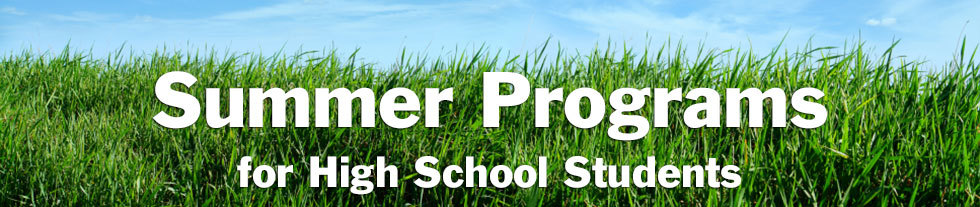# Summer Enrichment/Pre-College Programs

In addition to the virtual information sessions Northside Prep is offering after school daily and during the school day on Wednesdays (click HERE), the counseling department would like to share additional information about information sessions for summer enrichment opportunities.

Are you and your student interested in learning about enrichment opportunities or pre-college programs to engage in during the spring or summer? Would you like to build your resume and enhance your academic, personal, or social skills? If the answer is yes, consider reviewing the following resources to assist your family with identifying the best fit enrichment opportunities for your student:

 table div table+table+table+table+table+table+table+table+table+table+table+table+table+table+table+table+table+table+table+table+table+table+table+table+table+table+table+table+table+table+table+table+table+table+table+table+table+table+table+table+table+table+table+table+table+table div table{width:100%;padding:0}table div table+table+table+table+table+table+table+table+table+table+table+table+table+table+table+table+table+table+table+table+table+table+table+table+table+table+table+table+table+table+table+table+table+table+table+table+table+table+table+table+table+table+table+table+table+table div table img{width:96.23%;padding:0;float:none}table div table+table+table+table+table+table+table+table+table+table+table+table+table+table+table+table+table+table+table+table+table+table+table+table+table+table+table+table+table+table+table+table+table+table+table+table+table+table+table+table+table+table+table+table+table+table div table td{width:100%;padding:0 1.88% 18px}/* styles */# Chicago Teachers Union Scholarship

The Chicago Teachers Union Scholarship Committee is working hard to adapt and maintain our annual tradition of awarding scholarships to children of our members who are graduating and have plans to pursue post-secondary education. If you have a child who will be graduating from high school this year, NOW is the time for them to apply for a CTU/CTUF Scholarship! The deadline is May 10.

Scholarships in the amount of \$1,000 will be awarded to students to help defray the cost of post-secondary education in college or vocational programs. Details are available at www.ctulocal1.org/scholarship.

## Eligibility

Awards are entirely unrestricted as to race, religion, political affiliation, or choice of an accredited college, university, business, trade, or vocational school.

The applicant must be a child of a currently employed, active member in good standing of the Chicago Teachers Union, or a child of a deceased member who was in good standing with the Chicago Teachers Union at the time of death. They must also be a high school senior who will graduate this year before August 31. Special education students are eligible for all scholarships and are encouraged to apply.

## How to Apply

Please make certain that the application is completed in full, and that it is signed by the applicant AND the parent or guardian so that membership in good standing can be verified before presentation to the Scholarship Committee of the Chicago Teachers Union.

## Application Info

Scholarship winners will be notified by mail or email and invited to attend a special virtual reception and award ceremony with their families.

 table div table+table+table+table+table+table+table+table+table+table+table+table+table+table+table+table+table+table+table+table+table+table+table+table+table+table+table+table+table+table+table+table+table+table+table+table+table+table+table+table+table+table+table+table+table+table+table+table div table{width:100%;padding:0}table div table+table+table+table+table+table+table+table+table+table+table+table+table+table+table+table+table+table+table+table+table+table+table+table+table+table+table+table+table+table+table+table+table+table+table+table+table+table+table+table+table+table+table+table+table+table+table+table div table img{width:96.23%;padding:0;float:none}table div table+table+table+table+table+table+table+table+table+table+table+table+table+table+table+table+table+table+table+table+table+table+table+table+table+table+table+table+table+table+table+table+table+table+table+table+table+table+table+table+table+table+table+table+table+table+table+table div table td{width:100%;padding:0 1.88% 18px}/* styles */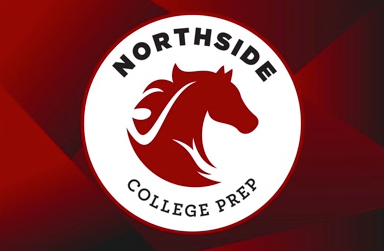# NCP Car Magnets and Window/Yard Signs Available

Friends of Northside is pleased to offer NCP car magnets for sale and to reopen orders for NCP yard/window signs. Show your school pride!

If you are interested, please click the button below. Each time we reach minimums for each item we will send for production and notify you of pickup timing. We anticipate two print runs for magnets and at least one for signs this school year. Go Mustangs!

Other swag is made available via the Saddle Shop directly via NCP; timing varies for their online pop-ups.

 table div table+table+table+table+table+table+table+table+table+table+table+table+table+table+table+table+table+table+table+table+table+table+table+table+table+table+table+table+table+table+table+table+table+table+table+table+table+table+table+table+table+table+table+table+table+table+table+table+table+table+table div table{width:100%;padding:0}table div table+table+table+table+table+table+table+table+table+table+table+table+table+table+table+table+table+table+table+table+table+table+table+table+table+table+table+table+table+table+table+table+table+table+table+table+table+table+table+table+table+table+table+table+table+table+table+table+table+table+table div table img{width:96.23%;padding:0;float:none}table div table+table+table+table+table+table+table+table+table+table+table+table+table+table+table+table+table+table+table+table+table+table+table+table+table+table+table+table+table+table+table+table+table+table+table+table+table+table+table+table+table+table+table+table+table+table+table+table+table+table+table div table td{width:100%;padding:0 1.88% 18px}/* styles */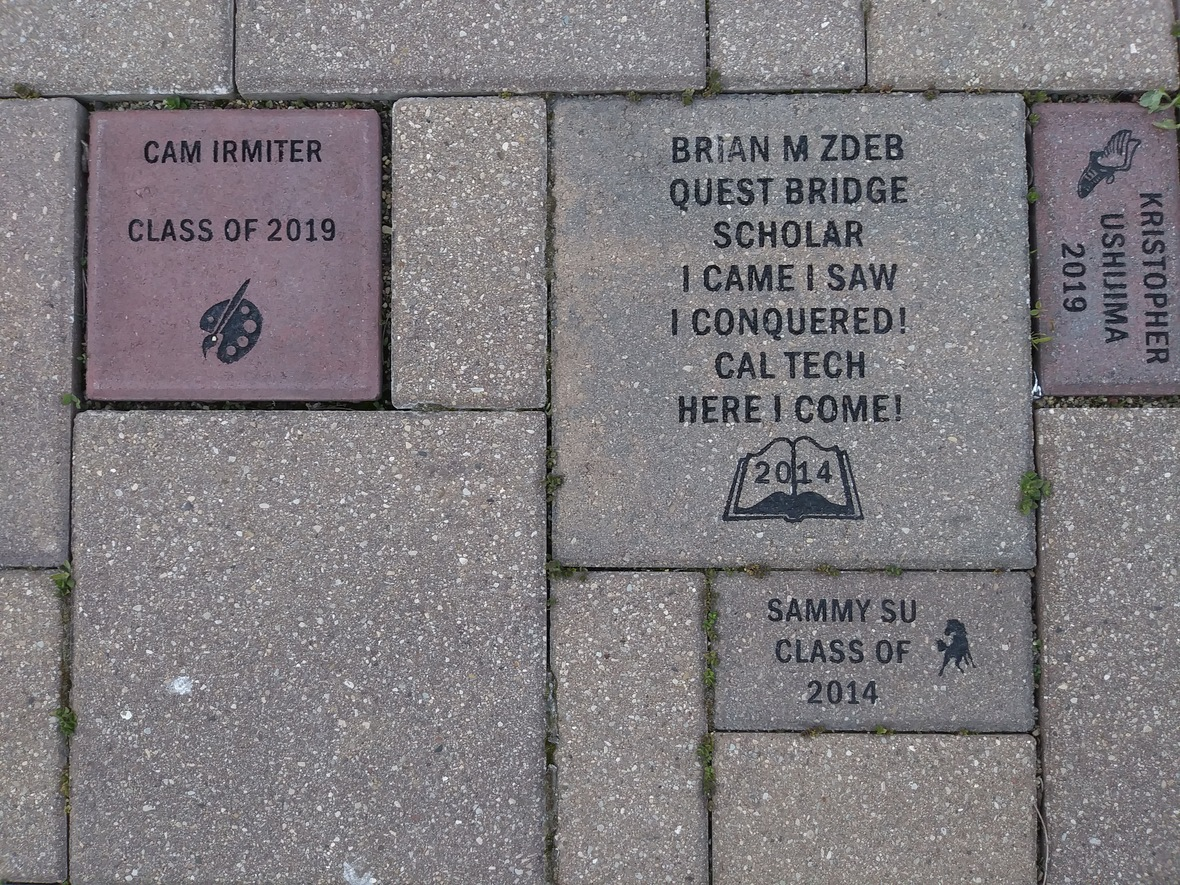Consider buying a custom NCP Brick for your child this year. Your brick, engraved with a personal message, will become a permanent part of the Northside College Prep Celestial Garden Walkway.

Your brick may be purchased in three sizes:

▪ 4" x 8" = \$50
▪ 8" x 8" = \$100
▪ 12" x 12" = \$150

Friends of Northside will provide a gift certificate acknowledging the purchase. Janet Jasmer will assist with the process and can answer any questions at jjasmer@atproperties.com.

 table div table+table+table+table+table+table+table+table+table+table+table+table+table+table+table+table+table+table+table+table+table+table+table+table+table+table+table+table+table+table+table+table+table+table+table+table+table+table+table+table+table+table+table+table+table+table+table+table+table+table+table+table+table div table{width:100%;padding:0}table div table+table+table+table+table+table+table+table+table+table+table+table+table+table+table+table+table+table+table+table+table+table+table+table+table+table+table+table+table+table+table+table+table+table+table+table+table+table+table+table+table+table+table+table+table+table+table+table+table+table+table+table+table div table img{width:96.23%;padding:0;float:none}table div table+table+table+table+table+table+table+table+table+table+table+table+table+table+table+table+table+table+table+table+table+table+table+table+table+table+table+table+table+table+table+table+table+table+table+table+table+table+table+table+table+table+table+table+table+table+table+table+table+table+table+table+table div table td{width:100%;padding:0 1.88% 18px}/* styles */table.module-53{width:68.87%;padding:0}table div table+table+table+table+table+table+table+table+table+table+table+table+table+table+table+table+table+table+table+table+table+table+table+table+table+table+table+table+table+table+table+table+table+table+table+table+table+table+table+table+table+table+table+table+table+table+table+table+table+table+table+table+table+table div table{width:68.87%;float:none;margin-left:auto;margin-right:auto;padding:0}table div table+table+table+table+table+table+table+table+table+table+table+table+table+table+table+table+table+table+table+table+table+table+table+table+table+table+table+table+table+table+table+table+table+table+table+table+table+table+table+table+table+table+table+table+table+table+table+table+table+table+table+table+table+table div table a{border:0 none;text-decoration:none}table div table+table+table+table+table+table+table+table+table+table+table+table+table+table+table+table+table+table+table+table+table+table+table+table+table+table+table+table+table+table+table+table+table+table+table+table+table+table+table+table+table+table+table+table+table+table+table+table+table+table+table+table+table+table div table img{width:100%!important;border:0 none;text-decoration:none}table div table+table+table+table+table+table+table+table+table+table+table+table+table+table+table+table+table+table+table+table+table+table+table+table+table+table+table+table+table+table+table+table+table+table+table+table+table+table+table+table+table+table+table+table+table+table+table+table+table+table+table+table+table+table div table td{width:100%;padding:0}/* styles */

# Order a Yearbook Today!

Interested in purchasing a copy of the 2020-2021 Stampede, NCP's yearbook? Click HERE to start your order! And if you didn't get a chance to purchase a copy of the 2019-2020 yearbook, you now have a change to buy one of those, too! Just email Ms. McCreedy at mkmccreedy@cps.edu after you've completed your 2020-2021 order.

Copies are now \$55.

 table div table+table+table+table+table+table+table+table+table+table+table+table+table+table+table+table+table+table+table+table+table+table+table+table+table+table+table+table+table+table+table+table+table+table+table+table+table+table+table+table+table+table+table+table+table+table+table+table+table+table+table+table+table+table+table+table+table div table{width:100%;padding:0}table div table+table+table+table+table+table+table+table+table+table+table+table+table+table+table+table+table+table+table+table+table+table+table+table+table+table+table+table+table+table+table+table+table+table+table+table+table+table+table+table+table+table+table+table+table+table+table+table+table+table+table+table+table+table+table+table+table div table img{width:96.23%;padding:0;float:none}table div table+table+table+table+table+table+table+table+table+table+table+table+table+table+table+table+table+table+table+table+table+table+table+table+table+table+table+table+table+table+table+table+table+table+table+table+table+table+table+table+table+table+table+table+table+table+table+table+table+table+table+table+table+table+table+table+table div table td{width:100%;padding:0 1.88% 18px}/* styles */table div table+table+table+table+table+table+table+table+table+table+table+table+table+table+table+table+table+table+table+table+table+table+table+table+table+table+table+table+table+table+table+table+table+table+table+table+table+table+table+table+table+table+table+table+table+table+table+table+table+table+table+table+table+table+table+table+table+table+table div table{width:100%;padding:0}table div table+table+table+table+table+table+table+table+table+table+table+table+table+table+table+table+table+table+table+table+table+table+table+table+table+table+table+table+table+table+table+table+table+table+table+table+table+table+table+table+table+table+table+table+table+table+table+table+table+table+table+table+table+table+table+table+table+table+table div table img{width:96.23%;padding:0;float:none}table div table+table+table+table+table+table+table+table+table+table+table+table+table+table+table+table+table+table+table+table+table+table+table+table+table+table+table+table+table+table+table+table+table+table+table+table+table+table+table+table+table+table+table+table+table+table+table+table+table+table+table+table+table+table+table+table+table+table+table div table td{width:100%;padding:0 1.88% 18px}/* styles */# Do you have news or team results to share with the Northside parent community?

 table div table+table+table+table+table+table+table+table+table+table+table+table+table+table+table+table+table+table+table+table+table+table+table+table+table+table+table+table+table+table+table+table+table+table+table+table+table+table+table+table+table+table+table+table+table+table+table+table+table+table+table+table+table+table+table+table+table+table+table+table+table div table{width:100%;padding:0}table div table+table+table+table+table+table+table+table+table+table+table+table+table+table+table+table+table+table+table+table+table+table+table+table+table+table+table+table+table+table+table+table+table+table+table+table+table+table+table+table+table+table+table+table+table+table+table+table+table+table+table+table+table+table+table+table+table+table+table+table+table div table img{width:96.23%;padding:0;float:none}table div table+table+table+table+table+table+table+table+table+table+table+table+table+table+table+table+table+table+table+table+table+table+table+table+table+table+table+table+table+table+table+table+table+table+table+table+table+table+table+table+table+table+table+table+table+table+table+table+table+table+table+table+table+table+table+table+table+table+table+table+table div table td{width:100%;padding:0 1.88% 18px}/* styles */# Support Northside - Shop AmazonSmile

AmazonSmile is a simple and automatic way to support Northside every time you shop.

Please note that our AmazonSmile name is Friends of "North" "Side" (North Side - two words at AmazonSmile).

 table div table+table+table+table+table+table+table+table+table+table+table+table+table+table+table+table+table+table+table+table+table+table+table+table+table+table+table+table+table+table+table+table+table+table+table+table+table+table+table+table+table+table+table+table+table+table+table+table+table+table+table+table+table+table+table+table+table+table+table+table+table+table+table div table{width:100%;padding:0}table div table+table+table+table+table+table+table+table+table+table+table+table+table+table+table+table+table+table+table+table+table+table+table+table+table+table+table+table+table+table+table+table+table+table+table+table+table+table+table+table+table+table+table+table+table+table+table+table+table+table+table+table+table+table+table+table+table+table+table+table+table+table+table div table img{width:96.23%;padding:0;float:none}table div table+table+table+table+table+table+table+table+table+table+table+table+table+table+table+table+table+table+table+table+table+table+table+table+table+table+table+table+table+table+table+table+table+table+table+table+table+table+table+table+table+table+table+table+table+table+table+table+table+table+table+table+table+table+table+table+table+table+table+table+table+table+table div table td{width:100%;padding:0 1.88% 18px}/* styles */# Follow Us on Social Media

 table div table+table+table+table+table+table+table+table+table+table+table+table+table+table+table+table+table+table+table+table+table+table+table+table+table+table+table+table+table+table+table+table+table+table+table+table+table+table+table+table+table+table+table+table+table+table+table+table+table+table+table+table+table+table+table+table+table+table+table+table+table+table+table+table+table div table{width:100%;padding:0}table div table+table+table+table+table+table+table+table+table+table+table+table+table+table+table+table+table+table+table+table+table+table+table+table+table+table+table+table+table+table+table+table+table+table+table+table+table+table+table+table+table+table+table+table+table+table+table+table+table+table+table+table+table+table+table+table+table+table+table+table+table+table+table+table+table div table img{width:96.23%;padding:0;float:none}table div table+table+table+table+table+table+table+table+table+table+table+table+table+table+table+table+table+table+table+table+table+table+table+table+table+table+table+table+table+table+table+table+table+table+table+table+table+table+table+table+table+table+table+table+table+table+table+table+table+table+table+table+table+table+table+table+table+table+table+table+table+table+table+table+table div table td{width:100%;padding:0 1.88% 18px}/* styles */table div table+table+table+table+table+table+table+table+table+table+table+table+table+table+table+table+table+table+table+table+table+table+table+table+table+table+table+table+table+table+table+table+table+table+table+table+table+table+table+table+table+table+table+table+table+table+table+table+table+table+table+table+table+table+table+table+table+table+table+table+table+table+table+table+table+table+table div table{width:100%;padding:0}table div table+table+table+table+table+table+table+table+table+table+table+table+table+table+table+table+table+table+table+table+table+table+table+table+table+table+table+table+table+table+table+table+table+table+table+table+table+table+table+table+table+table+table+table+table+table+table+table+table+table+table+table+table+table+table+table+table+table+table+table+table+table+table+table+table+table+table div table img{width:96.23%;padding:0;float:none}table div table+table+table+table+table+table+table+table+table+table+table+table+table+table+table+table+table+table+table+table+table+table+table+table+table+table+table+table+table+table+table+table+table+table+table+table+table+table+table+table+table+table+table+table+table+table+table+table+table+table+table+table+table+table+table+table+table+table+table+table+table+table+table+table+table+table+table div table td{width:100%;padding:0 1.88% 18px}/* styles */